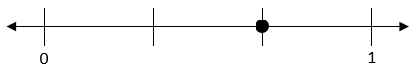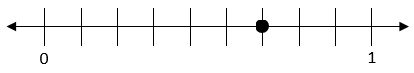# Fraction Equivalence and Ordering

## Objective

Recognize and generate equivalent fractions with smaller units using number lines.

## Common Core Standards

### Core Standards

?

• 4.NF.A.1 — Explain why a fraction a/b is equivalent to a fraction (n × a)/(n × b) by using visual fraction models, with attention to how the number and size of the parts differ even though the two fractions themselves are the same size. Use this principle to recognize and generate equivalent fractions.

?

• 3.NF.A.3

• 4.OA.A.1

• 4.OA.A.2

## Criteria for Success

?

1. Understand that equivalent fractions are fractions that refer to the same whole and are the same size.
2. Generate equivalent fractions with smaller units using visual models, namely number lines (MP.5).
3. Determine whether two fractions are equivalent using visual models and explain why the models demonstrate their equivalence (MP.3).

#### Fishtank Plus

• Problem Set
• Student Handout Editor
• Vocabulary Package

?

### Problem 1

1. What fraction represents the point shown on the number line below?1. What fraction represents the point shown on the number line below?1. Use the pictures to explain why the two fractions represented above are equivalent.

#### References

Illustrative Mathematics Explaining Fraction Equivalence with Pictures

Explaining Fraction Equivalence with Pictures, accessed on March 23, 2018, 8:50 a.m., is licensed by Illustrative Mathematics under either the CC BY 4.0 or CC BY-NC-SA 4.0. For further information, contact Illustrative Mathematics.

Modified by The Match Foundation, Inc.

### Problem 2

Find two fractions that are equivalent to each of the following:

a.    ${{1\over4}}$

b.    ${{2\over3}}$

c.    ${{5\over2}}$

### Problem 3

Determine whether the following are equivalent. Show or explain how you know.

a.   ${{2\over3}}$ and ${{8\over12}}$

b.   ${{3\over4}}$ and ${{8\over10}}$

c.    ${{5\over3}}$ and ${{10\over6}}$

## Problem Set & Homework

#### Discussion of Problem Set

• Even if you were not given the number lines in #1, how could you determine which answers were incorrect?
• What did you notice about the number lines in #2? What do you wonder?
• Which fractions were equivalent in #3? Which ones weren’t? How do you know?
• How did you decompose ${{2\over5}}$ into $4$ parts in #4b? What equivalent fraction did this produce?